# Resistor compensates for instrumentation-amp gain drift

## Analog Devices AD8295

Kenneth Gustafsson

EDN

Some instrumentation amplifiers use external resistors to set their gain. Unfortunately, the lack of temperature-coefficient matching between the external and the internal resistors results in a high gain drift. If, however, another on-chip resistor is available, you can use it to compensate for gain drift as a result of temperature.

As an example, Analog Devices’ AD8295 has a drift of as much as –50 ppm/°C, even if you use a zero-drift gain-setting resistor. In this Design Idea, you can compensate this drift with an extra zero-drift resistor in combination with an internal chip resistor.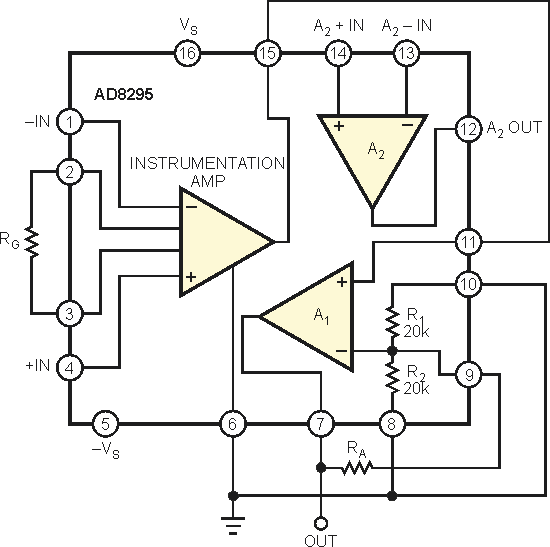Figure 1. In this configuration, the first-order drift of the gain cancels, and the gain splits equally between the instrumentation amplifier and A1.

The gain-set equation from the data sheet (Reference 1) is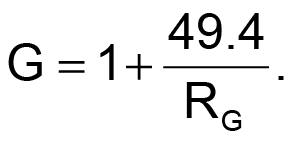where G is gain of the AD8295. From this gain-set equation, you can assume that the chip uses two 24.7-kΩ resistors with the external gain resistor, RG, to set the amplifier’s gain. The chip has two more 20-kΩ resistors. Because all of these chip resistors are of the same magnitude, they probably will have good temperature-coefficient matching, and you can use this matching for compensation. If the amplifier resistance, RA, and the gain resistor are zero-drift resistors (Figure 1), then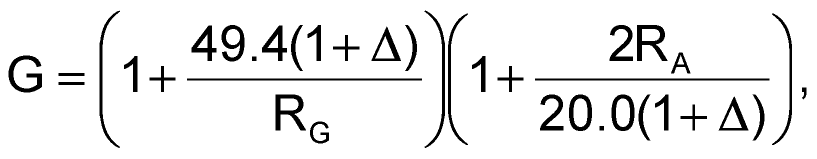where Δ is the drift of the internal matched resistors.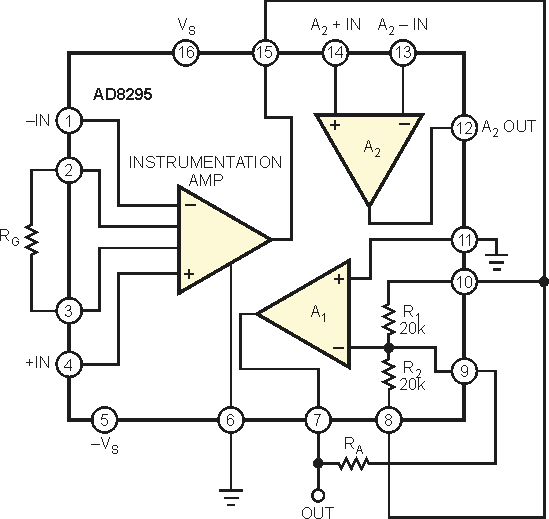Figure 2. For gain greater than 100, the amplifier resistance becomes greater than 90 kΩ, in which case you can use A1 in an inverting configuration with a gain of –1.

If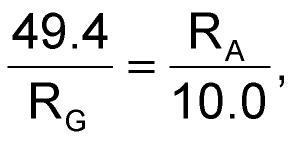then the first-order drift of the gain cancels, and the gain splits equally between the instrumentation amplifier and A1. Solving for RG and RA yields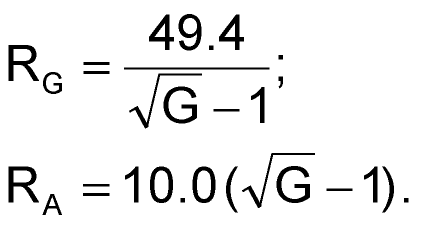For gain greater than 100, the amplifier resistance becomes greater than 90 kΩ, which can be problematic. In this case, you can use A1 in an inverting configuration with a gain of –1 (Figure 2). With an amplifier resistance of 10 kΩ,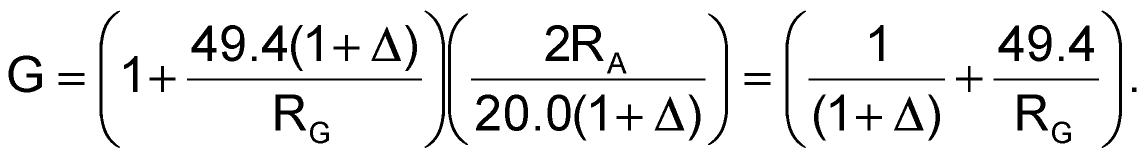This case sizes RG using a value from the data-sheet formula. If the gain is 50, the internal matching and the negative drift compensate the “49” part of the gain, and the “one” part is just the drift divided by 50 in the total gain, resulting in a typical figure of –1 ppm/°C. In both cases, the resulting gain temperature coefficient can be less than 5 ppm/°C, which is 10 times better than the original outcome.

## Materials on the topic

EDNYou may have to register before you can post comments and get full access to forum.
 User Name Remember Me? Password